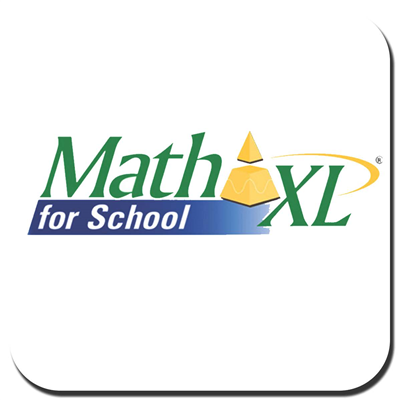# Foundational Skills in Mathematics 9-12 (#1200400)

This document was generated on CPALMS - www.cpalms.org
You are not viewing the current course, please click the current year’s tab.

#### Course Standards

Name Description
MA.4.NSO.2.1: Recall multiplication facts with factors up to 12 and related division facts with automaticity.
MA.5.GR.1.1: Classify triangles or quadrilaterals into different categories based on shared defining attributes. Explain why a triangle or quadrilateral would or would not belong to a category.
 Clarifications:Clarification 1: Triangles include scalene, isosceles, equilateral, acute, obtuse and right; quadrilaterals include parallelograms, rhombi, rectangles, squares and trapezoids.
MA.5.GR.1.2: Identify and classify three-dimensional figures into categories based on their defining attributes. Figures are limited to right pyramids, right prisms, right circular cylinders, right circular cones and spheres.
 Clarifications:Clarification 1: Defining attributes include the number and shape of faces, number and shape of bases, whether or not there is an apex, curved or straight edges and curved or flat faces.
MA.5.GR.2.1: Find the perimeter and area of a rectangle with fractional or decimal side lengths using visual models and formulas.
 Clarifications:Clarification 1: Instruction includes finding the area of a rectangle with fractional side lengths by tiling it with squares having unit fraction side lengths and showing that the area is the same as would be found by multiplying the side lengths. Clarification 2: Responses include the appropriate units in word form.
MA.6.AR.1.3: Evaluate algebraic expressions using substitution and order of operations.
 Clarifications:Clarification 1: Within this benchmark, the expectation is to perform all operations with integers. Clarification 2: Refer to Properties of Operations, Equality and Inequality (Appendix D).

 Examples:Evaluate the expression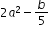, where a=-1 and b=15.
MA.6.AR.3.5: Solve mathematical and real-world problems involving ratios, rates and unit rates, including comparisons, mixtures, ratios of lengths and conversions within the same measurement system.
 Clarifications:Clarification 1: Instruction includes the use of tables, tape diagrams and number lines.
MA.6.DP.1.2: Given a numerical data set within a real-world context, find and interpret mean, median, mode and range.
 Clarifications:Clarification 1: Numerical data is limited to positive rational numbers.

 Examples:The data set {15,0,32,24,0,17,42,0,29,120,0,20}, collected based on minutes spent on homework, has a mode of 0.
MA.6.GR.1.3: Solve mathematical and real-world problems by plotting points on a coordinate plane, including finding the perimeter or area of a rectangle.
 Clarifications:Clarification 1: Instruction includes finding distances between points, computing dimensions of a rectangle or determining a fourth vertex of a rectangle. Clarification 2: Problems involving rectangles are limited to cases where the sides are parallel to the axes.
MA.6.GR.2.2: Solve mathematical and real-world problems involving the area of quadrilaterals and composite figures by decomposing them into triangles or rectangles.
 Clarifications:Clarification 1: Problem types include finding area of composite shapes and determining missing dimensions. Clarification 2: Within this benchmark, the expectation is to know from memory a formula for the area of a rectangle and triangle. Clarification 3: Dimensions are limited to positive rational numbers.
MA.6.NSO.1.1: Extend previous understanding of numbers to define rational numbers. Plot, order and compare rational numbers.
 Clarifications:Clarification 1: Within this benchmark, the expectation is to plot, order and compare positive and negative rational numbers when given in the same form and to plot, order and compare positive rational numbers when given in different forms (fraction, decimal, percentage). Clarification 2: Within this benchmark, the expectation is to use symbols (<, > or =).
MA.6.NSO.4.1: Apply and extend previous understandings of operations with whole numbers to add and subtract integers with procedural fluency.
 Clarifications:Clarification 1: Instruction begins with the use of manipulatives, models and number lines working towards becoming procedurally fluent by the end of grade 6. Clarification 2: Instruction focuses on the inverse relationship between the operations of addition and subtraction. If p and q are integers, then p-q=p+(-q) and p+q=p-(-q).
MA.6.NSO.4.2: Apply and extend previous understandings of operations with whole numbers to multiply and divide integers with procedural fluency.
 Clarifications:Clarification 1: Instruction includes the use of models and number lines and the inverse relationship between multiplication and division, working towards becoming procedurally fluent by the end of grade 6. Clarification 2: Instruction focuses on the understanding that integers can be divided, provided that the divisor is not zero, and every quotient of integers (with non-zero divisor) is a rational number. If p and q are integers where q≠0, then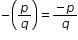,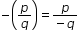and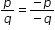.
MA.7.AR.3.3: Solve mathematical and real-world problems involving the conversion of units across different measurement systems.
 Examples:Clarification 1: Problem types are limited to length, area, weight, mass, volume and money.
MA.7.AR.4.5: Solve real-world problems involving proportional relationships.
 Examples:Gordy is taking a trip from Tallahassee, FL to Portland, Maine which is about 1,407 miles. On average his SUV gets 23.1 miles per gallon on the highway and his gas tanks holds 17.5 gallons. If Gordy starts with a full tank of gas, how many times will he be required to fill the gas tank?
MA.7.DP.1.2: Given two numerical or graphical representations of data, use the measure(s) of center and measure(s) of variability to make comparisons, interpret results and draw conclusions about the two populations.
 Clarifications:Clarification 1: Graphical representations are limited to histograms, line plots, box plots and stem-and-leaf plots. Clarification 2: The measure of center is limited to mean and median. The measure of variation is limited to range and interquartile range.
MA.7.DP.1.5: Given a real-world numerical or categorical data set, choose and create an appropriate graphical representation.
 Clarifications:Clarification 1: Graphical representations are limited to histograms, bar charts, circle graphs, line plots, box plots and stem-and-leaf plots.
MA.7.DP.2.4: Use a simulation of a simple experiment to find experimental probabilities and compare them to theoretical probabilities.
 Clarifications:Clarification 1: Instruction includes representing probability as a fraction, percentage or decimal. Clarification 2: Instruction includes recognizing that experimental probabilities may differ from theoretical probabilities due to random variation. As the number of repetitions increases experimental probabilities will typically better approximate the theoretical probabilities. Clarification 3: Experiments include tossing a fair coin, rolling a fair die, picking a card randomly from a deck, picking marbles randomly from a bag and spinning a fair spinner.

 Examples:Investigate whether a coin is fair by tossing it 1,000 times and comparing the percentage of heads to the theoretical probability 0.5.
MA.7.NSO.1.2: Rewrite rational numbers in different but equivalent forms including fractions, mixed numbers, repeating decimals and percentages to solve mathematical and real-world problems.
 Examples:Justin is solving a problem where he computes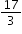and his calculator gives him the answer 5.6666666667. Justin makes the statement that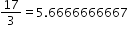; is he correct?
MA.7.NSO.2.2: Add, subtract, multiply and divide rational numbers with procedural fluency.
MA.7.NSO.2.3: Solve real-world problems involving any of the four operations with rational numbers.
 Clarifications:Clarification 1: Instruction includes using one or more operations to solve problems.
MA.8.AR.1.2: Apply properties of operations to multiply two linear expressions with rational coefficients.
 Clarifications:Clarification 1: Problems are limited to products where at least one of the factors is a monomial. Clarification 2: Refer to Properties of Operations, Equality and Inequality (Appendix D).

 Examples:The product of (1.1+x) and (-2.3x) can be expressed as -2.53x-2.3x² or -2.3x²-2.53x.
MA.8.AR.1.3: Rewrite the sum of two algebraic expressions having a common monomial factor as a common factor multiplied by the sum of two algebraic expressions.
 Examples:The expression 99x-11x³ can be rewritten as 11x(9-x²) or as -11x(-9+x² ).
MA.8.DP.2.3: Solve real-world problems involving probabilities related to single or repeated experiments, including making predictions based on theoretical probability.
 Clarifications:Clarification 1: Instruction includes making connections to proportional relationships and representing probability as a fraction, percentage or decimal. Clarification 2: Experiments to be repeated are limited to tossing a fair coin, rolling a fair die, picking a card randomly from a deck with replacement, picking marbles randomly from a bag with replacement and spinning a fair spinner. Clarification 3: Repetition of experiments is limited to two times except for tossing a coin.

 Examples:Example: If Gabriella rolls a fair die 300 times, she can predict that she will roll a 3 approximately 50 times since the theoretical probability is. Example: Sandra performs an experiment where she flips a coin three times. She finds the theoretical probability of landing on exactly one head as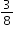. If she performs this experiment 50 times (for a total of 150 flips), predict the number of repetitions of the experiment that will result in exactly one of the three flips landing on heads.
MA.8.NSO.1.1: Extend previous understanding of rational numbers to define irrational numbers within the real number system. Locate an approximate value of a numerical expression involving irrational numbers on a number line.
 Clarifications:Clarification 1: Instruction includes the use of number line and rational number approximations, and recognizing pi (π) as an irrational number. Clarification 2: Within this benchmark, the expectation is to approximate numerical expressions involving one arithmetic operation and estimating square roots or pi (π).

 Examples:Within the expression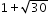, the irrational number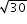can be estimated to be between 5 and 6 because 30 is between 25 and 36. By considering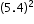and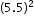, a closer approximation foris 5.5. So, the expressionis equivalent to about 6.5.
MA.8.NSO.1.2: Plot, order and compare rational and irrational numbers, represented in various forms.
 Clarifications:Clarification 1: Within this benchmark, it is not the expectation to work with the number e. Clarification 2: Within this benchmark, the expectation is to plot, order and compare square roots and cube roots. Clarification 3: Within this benchmark, the expectation is to use symbols (<, > or =).
MA.8.NSO.1.7: Solve multi-step mathematical and real-world problems involving the order of operations with rational numbers including exponents and radicals.
 Clarifications:Clarification 1: Multi-step expressions are limited to 6 or fewer steps. Clarification 2: Within this benchmark, the expectation is to simplify radicals by factoring square roots of perfect squares up to 225 and cube roots of perfect cubes from -125 to 125.

 Examples:The expression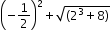is equivalent to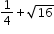which is equivalent to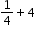which is equivalent to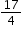.
MA.912.AR.1.1: Identify and interpret parts of an equation or expression that represent a quantity in terms of a mathematical or real-world context, including viewing one or more of its parts as a single entity.
 Clarifications:Clarification 1: Parts of an expression include factors, terms, constants, coefficients and variables. Clarification 2: Within the Mathematics for Data and Financial Literacy course, problem types focus on money and business.

 Examples:Algebra 1 Example: Derrick is using the formula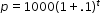to make a prediction about the camel population in Australia. He identifies the growth factor as (1+.1), or 1.1, and states that the camel population will grow at an annual rate of 10% per year.Example: The expression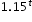can be rewritten as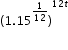which is approximately equivalent to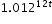. This latter expression reveals the approximate equivalent monthly interest rate of 1.2% if the annual rate is 15%.
MA.912.AR.1.2: Rearrange equations or formulas to isolate a quantity of interest.
 Clarifications:Clarification 1: Instruction includes using formulas for temperature, perimeter, area and volume; using equations for linear (standard, slope-intercept and point-slope forms) and quadratic (standard, factored and vertex forms) functions. Clarification 2: Within the Mathematics for Data and Financial Literacy course, problem types focus on money and business.

 Examples:Algebra 1 Example: The Ideal Gas Law PV = nRT can be rearranged as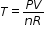to isolate temperature as the quantity of interest. Example: Given the Compound Interest formula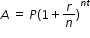, solve for P. Mathematics for Data and Financial Literacy Honors Example: Given the Compound Interest formula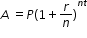, solve for t.
MA.912.AR.1.3: Add, subtract and multiply polynomial expressions with rational number coefficients.
 Clarifications:Clarification 1: Instruction includes an understanding that when any of these operations are performed with polynomials the result is also a polynomial.Clarification 2: Within the Algebra 1 course, polynomial expressions are limited to 3 or fewer terms.
MA.912.AR.1.4: Divide a polynomial expression by a monomial expression with rational number coefficients.
 Clarifications:Clarification 1: Within the Algebra 1 course, polynomial expressions are limited to 3 or fewer terms.
MA.912.AR.1.5: Divide polynomial expressions using long division, synthetic division or algebraic manipulation.
MA.912.AR.1.7: Rewrite a polynomial expression as a product of polynomials over the real number system.
 Clarifications:Clarification 1: Within the Algebra 1 course, polynomial expressions are limited to 4 or fewer terms with integer coefficients.

 Examples:Example: The expression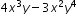is equivalent to the factored form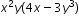. Example: The expression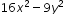is equivalent to the factored form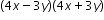.
MA.912.AR.1.9: Apply previous understanding of rational number operations to add, subtract, multiply and divide rational algebraic expressions.
 Clarifications:Clarification 1: Instruction includes the connection to fractions and common denominators.
MA.912.AR.2.1: Given a real-world context, write and solve one-variable multi-step linear equations.
MA.912.AR.2.2: Write a linear two-variable equation to represent the relationship between two quantities from a graph, a written description or a table of values within a mathematical or real-world context.
 Clarifications:Clarification 1: Instruction includes the use of standard form, slope-intercept form and point-slope form, and the conversion between these forms.
MA.912.AR.2.3: Write a linear two-variable equation for a line that is parallel or perpendicular to a given line and goes through a given point.
 Clarifications:Clarification 1: Instruction focuses on recognizing that perpendicular lines have slopes that when multiplied result in -1 and that parallel lines have slopes that are the same. Clarification 2: Instruction includes representing a line with a pair of points on the coordinate plane or with an equation. Clarification 3: Problems include cases where one variable has a coefficient of zero.
MA.912.AR.2.4: Given a table, equation or written description of a linear function, graph that function, and determine and interpret its key features.
 Clarifications:Clarification 1: Key features are limited to domain, range, intercepts and rate of change. Clarification 2: Instruction includes the use of standard form, slope-intercept form and point-slope form.Clarification 3: Instruction includes cases where one variable has a coefficient of zero.Clarification 4: Instruction includes representing the domain and range with inequality notation, interval notation or set-builder notation.Clarification 5: Within the Algebra 1 course, notations for domain and range are limited to inequality and set-builder notations.
MA.912.AR.2.5: Solve and graph mathematical and real-world problems that are modeled with linear functions. Interpret key features and determine constraints in terms of the context.
 Clarifications:Clarification 1: Key features are limited to domain, range, intercepts and rate of change.Clarification 2: Instruction includes the use of standard form, slope-intercept form and point-slope form.Clarification 3: Instruction includes representing the domain, range and constraints with inequality notation, interval notation or set-builder notation.Clarification 4: Within the Algebra 1 course, notations for domain, range and constraints are limited to inequality and set-builder.Clarification 5: Within the Mathematics for Data and Financial Literacy course, problem types focus on money and business.

 Examples:Algebra 1 Example: Lizzy’s mother uses the function C(p)=450+7.75p, where C(p) represents the total cost of a rental space and p is the number of people attending, to help budget Lizzy’s 16th birthday party. Lizzy’s mom wants to spend no more than \$850 for the party. Graph the function in terms of the context.
MA.912.AR.2.6: Given a mathematical or real-world context, write and solve one-variable linear inequalities, including compound inequalities. Represent solutions algebraically or graphically.
 Examples:Algebra 1 Example: The compound inequality 2x≤5x+1<4 is equivalent to -1≤3x and 5x<3, which is equivalent to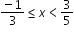.
MA.912.AR.2.7: Write two-variable linear inequalities to represent relationships between quantities from a graph or a written description within a mathematical or real-world context.
 Clarifications:Clarification 1: Instruction includes the use of standard form, slope-intercept form and point-slope form and any inequality symbol can be represented. Clarification 2: Instruction includes cases where one variable has a coefficient of zero.
MA.912.AR.2.8: Given a mathematical or real-world context, graph the solution set to a two-variable linear inequality.
 Clarifications:Clarification 1: Instruction includes the use of standard form, slope-intercept form and point-slope form and any inequality symbol can be represented. Clarification 2: Instruction includes cases where one variable has a coefficient of zero.
MA.912.AR.3.1: Given a mathematical or real-world context, write and solve one-variable quadratic equations over the real number system.
 Clarifications:Clarification 1: Within the Algebra 1 course, instruction includes the concept of non-real answers, without determining non-real solutions. Clarification 2: Within this benchmark, the expectation is to solve by factoring techniques, taking square roots, the quadratic formula and completing the square.
MA.912.AR.3.2: Given a mathematical or real-world context, write and solve one-variable quadratic equations over the real and complex number systems.
 Clarifications:Clarification 1: Within this benchmark, the expectation is to solve by factoring techniques, taking square roots, the quadratic formula and completing the square.
MA.912.AR.3.5: Given the x-intercepts and another point on the graph of a quadratic function, write the equation for the function.
MA.912.AR.3.6: Given an expression or equation representing a quadratic function, determine the vertex and zeros and interpret them in terms of a real-world context.
MA.912.AR.3.7: Given a table, equation or written description of a quadratic function, graph that function, and determine and interpret its key features.
 Clarifications:Clarification 1: Key features are limited to domain; range; intercepts; intervals where the function is increasing, decreasing, positive or negative; end behavior; vertex; and symmetry.Clarification 2: Instruction includes the use of standard form, factored form and vertex form, and sketching a graph using the zeros and vertex.Clarification 3: Instruction includes representing the domain and range with inequality notation, interval notation or set-builder notation.Clarification 4: Within the Algebra 1 course, notations for domain and range are limited to inequality and set-builder.
MA.912.AR.3.8: Solve and graph mathematical and real-world problems that are modeled with quadratic functions. Interpret key features and determine constraints in terms of the context.
 Clarifications:Clarification 1: Key features are limited to domain; range; intercepts; intervals where the function is increasing, decreasing, positive or negative; end behavior; vertex; and symmetry.Clarification 2: Instruction includes the use of standard form, factored form and vertex form.Clarification 3: Instruction includes representing the domain, range and constraints with inequality notation, interval notation or set-builder notation.Clarification 4: Within the Algebra 1 course, notations for domain, range and constraints are limited to inequality and set-builder.

 Examples:Algebra 1 Example: The value of a classic car produced in 1972 can be modeled by the function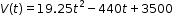, where t is the number of years since 1972. In what year does the car’s value start to increase?
MA.912.AR.3.9: Given a mathematical or real-world context, write two-variable quadratic inequalities to represent relationships between quantities from a graph or a written description.
 Clarifications:Clarification 1: Instruction includes the use of standard form, factored form and vertex form where any inequality symbol can be represented.
MA.912.AR.3.10: Given a mathematical or real-world context, graph the solution set to a two-variable quadratic inequality.
 Clarifications:Clarification 1: Instruction includes the use of standard form, factored form and vertex form where any inequality symbol can be represented.
MA.912.AR.4.1: Given a mathematical or real-world context, write and solve one-variable absolute value equations.
MA.912.AR.4.2: Given a mathematical or real-world context, write and solve one-variable absolute value inequalities. Represent solutions algebraically or graphically.
MA.912.AR.4.3: Given a table, equation or written description of an absolute value function, graph that function and determine its key features.
 Clarifications:Clarification 1: Key features are limited to domain; range; intercepts; intervals where the function is increasing, decreasing, positive or negative; vertex; end behavior and symmetry. Clarification 2: Instruction includes representing the domain and range with inequality notation, interval notation or set-builder notation.Clarification 3: Within the Algebra 1 course, notations for domain and range are limited to inequality and set-builder.
MA.912.AR.4.4: Solve and graph mathematical and real-world problems that are modeled with absolute value functions. Interpret key features and determine constraints in terms of the context.
 Clarifications:Clarification 1: Key features are limited to domain; range; intercepts; intervals where the function is increasing, decreasing, positive or negative; vertex; end behavior and symmetry.Clarification 2: Instruction includes representing the domain, range and constraints with inequality notation, interval notation or set-builder notation.
MA.912.AR.5.1: Solve one-variable exponential equations using the properties of exponents.
MA.912.AR.5.3: Given a mathematical or real-world context, classify an exponential function as representing growth or decay.
 Clarifications:Clarification 1: Within the Algebra 1 course, exponential functions are limited to the forms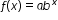, where b is a whole number greater than 1 or a unit fraction, or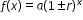, where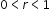.
MA.912.AR.5.4: Write an exponential function to represent a relationship between two quantities from a graph, a written description or a table of values within a mathematical or real-world context.
 Clarifications:Clarification 1: Within the Algebra 1 course, exponential functions are limited to the forms, where b is a whole number greater than 1 or a unit fraction, or, where.Clarification 2: Within the Algebra 1 course, tables are limited to having successive nonnegative integer inputs so that the function may be determined by finding ratios between successive outputs.
MA.912.AR.5.6: Given a table, equation or written description of an exponential function, graph that function and determine its key features.
 Clarifications:Clarification 1: Key features are limited to domain; range; intercepts; intervals where the function is increasing, decreasing, positive or negative; constant percent rate of change; end behavior and asymptotes. Clarification 2: Instruction includes representing the domain and range with inequality notation, interval notation or set-builder notation. Clarification 3: Within the Algebra 1 course, notations for domain and range are limited to inequality and set-builder. Clarification 4: Within the Algebra 1 course, exponential functions are limited to the forms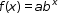, where b is a whole number greater than 1 or a unit fraction or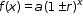, where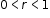.
MA.912.AR.5.8: Given a table, equation or written description of a logarithmic function, graph that function and determine its key features.
 Clarifications:Clarification 1: Key features are limited to domain; range; intercepts; intervals where the function is increasing, decreasing, positive or negative; end behavior; and asymptotes. Clarification 2: Instruction includes representing the domain and range with inequality notation, interval notation or set-builder notation.
MA.912.AR.6.1: Given a mathematical or real-world context, when suitable factorization is possible, solve one-variable polynomial equations of degree 3 or higher over the real and complex number systems.
MA.912.AR.6.5: Sketch a rough graph of a polynomial function of degree 3 or higher using zeros, multiplicity and knowledge of end behavior.
MA.912.AR.7.1: Solve one-variable radical equations. Interpret solutions as viable in terms of context and identify any extraneous solutions.
MA.912.AR.7.2: Given a table, equation or written description of a square root or cube root function, graph that function and determine its key features.
 Clarifications:Clarification 1: Key features are limited to domain; range; intercepts; intervals where the function is increasing, decreasing, positive or negative; end behavior; and relative maximums and minimums. Clarification 2: Instruction includes representing the domain and range with inequality notation, interval notation or set-builder notation.
MA.912.AR.8.1: Write and solve one-variable rational equations. Interpret solutions as viable in terms of the context and identify any extraneous solutions.
 Clarifications:Clarification 1: Within the Algebra 2 course, numerators and denominators are limited to linear and quadratic expressions.
MA.912.AR.8.2: Given a table, equation or written description of a rational function, graph that function and determine its key features.
 Clarifications:Clarification 1: Key features are limited to domain; range; intercepts; intervals where the function is increasing, decreasing, positive or negative; end behavior; and asymptotes.Clarification 2: Instruction includes representing the domain and range with inequality notation, interval notation or set-builder notation.Clarification 3: Within the Algebra 2 course, numerators and denominators are limited to linear and quadratic expressions.
MA.912.AR.9.1: Given a mathematical or real-world context, write and solve a system of two-variable linear equations algebraically or graphically.
 Clarifications:Clarification 1: Within this benchmark, the expectation is to solve systems using elimination, substitution and graphing.Clarification 2: Within the Algebra 1 course, the system is limited to two equations.
MA.912.AR.9.4: Graph the solution set of a system of two-variable linear inequalities.
 Clarifications:Clarification 1: Instruction includes cases where one variable has a coefficient of zero. Clarification 2: Within the Algebra 1 course, the system is limited to two inequalities.
MA.912.AR.9.6: Given a real-world context, represent constraints as systems of linear equations or inequalities. Interpret solutions to problems as viable or non-viable options.
 Clarifications:Clarification 1: Instruction focuses on analyzing a given function that models a real-world situation and writing constraints that are represented as linear equations or linear inequalities.
MA.912.AR.9.10: Solve and graph mathematical and real-world problems that are modeled with piecewise functions. Interpret key features and determine constraints in terms of the context.
 Clarifications:Clarification 1: Key features are limited to domain, range, intercepts, asymptotes and end behavior.Clarification 2: Instruction includes representing the domain, range and constraints with inequality notation, interval notation or set-builder notation.

 Examples:A mechanic wants to place an ad in his local newspaper. The cost, in dollars, of an ad x inches long is given by the following piecewise function. Find the cost of an ad that would be 16 inches long.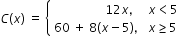MA.912.AR.10.1: Given a mathematical or real-world context, write and solve problems involving arithmetic sequences.
 Examples:Tara is saving money to move out of her parent’s house. She opens the account with \$250 and puts \$100 into a savings account every month after that. Write the total amount of money she has in her account after each month as a sequence. In how many months will she have at least \$3,000?
MA.912.AR.10.2: Given a mathematical or real-world context, write and solve problems involving geometric sequences.
 Examples:A bacteria in a Petri dish initially covers 2 square centimeters. The bacteria grows at a rate of 2.6% every day. Determine the geometric sequence that describes the area covered by the bacteria after 0,1,2,3… days. Determine using technology, how many days it would take the bacteria to cover 10 square centimeters.
MA.912.DP.1.1: Given a set of data, select an appropriate method to represent the data, depending on whether it is numerical or categorical data and on whether it is univariate or bivariate.
 Clarifications:Clarification 1: Instruction includes discussions regarding the strengths and weaknesses of each data display. Clarification 2: Numerical univariate includes histograms, stem-and-leaf plots, box plots and line plots; numerical bivariate includes scatter plots and line graphs; categorical univariate includes bar charts, circle graphs, line plots, frequency tables and relative frequency tables; and categorical bivariate includes segmented bar charts, joint frequency tables and joint relative frequency tables. Clarification 3: Instruction includes the use of appropriate units and labels and, where appropriate, using technology to create data displays.
MA.912.DP.1.2: Interpret data distributions represented in various ways. State whether the data is numerical or categorical, whether it is univariate or bivariate and interpret the different components and quantities in the display.
 Clarifications:Clarification 1: Within the Probability and Statistics course, instruction includes the use of spreadsheets and technology.
MA.912.DP.1.3: Explain the difference between correlation and causation in the contexts of both numerical and categorical data.
 Examples:Algebra 1 Example: There is a strong positive correlation between the number of Nobel prizes won by country and the per capita chocolate consumption by country. Does this mean that increased chocolate consumption in America will increase the United States of America’s chances of a Nobel prize winner?
MA.912.DP.1.4: Estimate a population total, mean or percentage using data from a sample survey; develop a margin of error through the use of simulation.
 Clarifications:Clarification 1: Within the Algebra 1 course, the margin of error will be given.

 Examples:Algebra 1 Example: Based on a survey of 100 households in Twin Lakes, the newspaper reports that the average number of televisions per household is 3.5 with a margin of error of ±0.6. The actual population mean can be estimated to be between 2.9 and 4.1 television per household. Since there are 5,500 households in Twin Lakes the estimated number of televisions is between 15,950 and 22,550.
MA.912.DP.2.1: For two or more sets of numerical univariate data, calculate and compare the appropriate measures of center and measures of variability, accounting for possible effects of outliers. Interpret any notable features of the shape of the data distribution.
 Clarifications:Clarification 1: The measure of center is limited to mean and median. The measure of variation is limited to range, interquartile range, and standard deviation.Clarification 2: Shape features include symmetry or skewness and clustering.Clarification 3: Within the Probability and Statistics course, instruction includes the use of spreadsheets and technology.
MA.912.DP.2.4: Fit a linear function to bivariate numerical data that suggests a linear association and interpret the slope and y-intercept of the model. Use the model to solve real-world problems in terms of the context of the data.
 Clarifications:Clarification 1: Instruction includes fitting a linear function both informally and formally with the use of technology.Clarification 2: Problems include making a prediction or extrapolation, inside and outside the range of the data, based on the equation of the line of fit.
MA.912.DP.2.5: Given a scatter plot that represents bivariate numerical data, assess the fit of a given linear function by plotting and analyzing residuals.
 Clarifications:Clarification 1: Within the Algebra 1 course, instruction includes determining the number of positive and negative residuals; the largest and smallest residuals; and the connection between outliers in the data set and the corresponding residuals.
MA.912.DP.2.6: Given a scatter plot with a line of fit and residuals, determine the strength and direction of the correlation. Interpret strength and direction within a real-world context.
 Clarifications:Clarification 1: Instruction focuses on determining the direction by analyzing the slope and informally determining the strength by analyzing the residuals.
MA.912.DP.3.1: Construct a two-way frequency table summarizing bivariate categorical data. Interpret joint and marginal frequencies and determine possible associations in terms of a real-world context.
Examples:
Algebra 1 Example: Complete the frequency table below.

 Has an A in math Doesn't have an A in math Total Plays an instrument 20 90 Doesn't play an instrument 20 Total 350

Using the information in the table, it is possible to determine that the second column contains the numbers 70 and 240. This means that there are 70 students who play an instrument but do not have an A in math and the total number of students who play an instrument is 90. The ratio of the joint frequencies in the first column is 1 to 1 and the ratio in the second column is 7 to 24, indicating a strong positive association between playing an instrument and getting an A in math.

MA.912.DP.3.2: Given marginal and conditional relative frequencies, construct a two-way relative frequency table summarizing categorical bivariate data.
 Clarifications:Clarification 1: Construction includes cases where not all frequencies are given but enough are provided to be able to construct a two-way relative frequency table. Clarification 2: Instruction includes the use of a tree diagram when calculating relative frequencies to construct tables.

Examples:
Algebra 1 Example: A study shows that 9% of the population have diabetes and 91% do not. The study also shows that 95% of the people who do not have diabetes, test negative on a diabetes test while 80% who do have diabetes, test positive. Based on the given information, the following relative frequency table can be constructed.
 Positive Negative Total Has diabetes 7.2% 1.8% 9% Doesn't have diabetes 4.55% 86.45% 91%

MA.912.DP.3.3: Given a two-way relative frequency table or segmented bar graph summarizing categorical bivariate data, interpret joint, marginal and conditional relative frequencies in terms of a real-world context.
 Clarifications:Clarification 1: Instruction includes problems involving false positive and false negatives.

Examples:
Algebra 1 Example: Given the relative frequency table below, the ratio of true positives to false positives can be determined as 7.2 to 4.55, which is about 3 to 2, meaning that a randomly selected person who tests positive for diabetes is about 50% more likely to have diabetes than not have it.

 Positive Negative Total Has diabetes 7.2% 1.8% 9% Doesn't have diabetes 4.55% 86.45% 91%

MA.912.F.1.1: Given an equation or graph that defines a function, determine the function type. Given an input-output table, determine a function type that could represent it.
 Clarifications:Clarification 1: Within the Algebra 1 course, functions represented as tables are limited to linear, quadratic and exponential. Clarification 2: Within the Algebra 1 course, functions represented as equations or graphs are limited to vertical or horizontal translations or reflections over the x-axis of the following parent functions: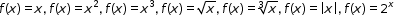and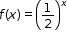.
MA.912.F.1.2: Given a function represented in function notation, evaluate the function for an input in its domain. For a real-world context, interpret the output.
 Clarifications:Clarification 1: Problems include simple functions in two-variables, such as f(x,y)=3x-2y. Clarification 2: Within the Algebra 1 course, functions are limited to one-variable such as f(x)=3x.

 Examples:Algebra 1 Example: The function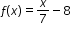models Alicia’s position in miles relative to a water stand x minutes into a marathon. Evaluate and interpret for a quarter of an hour into the race.
MA.912.F.1.3: Calculate and interpret the average rate of change of a real-world situation represented graphically, algebraically or in a table over a specified interval.
 Clarifications:Clarification 1: Instruction includes making the connection to determining the slope of a particular line segment.
MA.912.F.1.5: Compare key features of linear functions each represented algebraically, graphically, in tables or written descriptions.
 Clarifications:Clarification 1: Key features are limited to domain; range; intercepts; slope and end behavior.
MA.912.F.1.6: Compare key features of linear and nonlinear functions each represented algebraically, graphically, in tables or written descriptions.
 Clarifications:Clarification 1: Key features are limited to domain; range; intercepts; intervals where the function is increasing, decreasing, positive or negative; end behavior and asymptotes. Clarification 2: Within the Algebra 1 course, functions other than linear, quadratic or exponential must be represented graphically. Clarification 3: Within the Algebra 1 course, instruction includes verifying that a quantity increasing exponentially eventually exceeds a quantity increasing linearly or quadratically.
MA.912.F.1.8: Determine whether a linear, quadratic or exponential function best models a given real-world situation.
 Clarifications:Clarification 1: Instruction includes recognizing that linear functions model situations in which a quantity changes by a constant amount per unit interval; that quadratic functions model situations in which a quantity increases to a maximum, then begins to decrease or a quantity decreases to a minimum, then begins to increase; and that exponential functions model situations in which a quantity grows or decays by a constant percent per unit interval. Clarification 2: Within this benchmark, the expectation is to identify the type of function from a written description or table.
MA.912.F.2.1: Identify the effect on the graph or table of a given function after replacing f(x) by f(x)+k,kf(x), f(kx) and f(x+k) for specific values of k.
 Clarifications:Clarification 1: Within the Algebra 1 course, functions are limited to linear, quadratic and absolute value.Clarification 2: Instruction focuses on including positive and negative values for k.
MA.912.F.2.3: Given the graph or table of f(x) and the graph or table of f(x)+k,kf(x), f(kx) and f(x+k), state the type of transformation and find the value of the real number k.
 Clarifications:Clarification 1: Within the Algebra 1 course, functions are limited to linear, quadratic and absolute value.
MA.912.F.3.1: Given a mathematical or real-world context, combine two functions, limited to linear and quadratic, using arithmetic operations. When appropriate, include domain restrictions for the new function.
 Clarifications:Clarification 1: Instruction includes representing domain restrictions with inequality notation, interval notation or set-builder notation.Clarification 2: Within the Algebra 1 Honors course, notations for domain and range are limited to inequality and set-builder.

 Examples:The quotient of the functions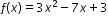and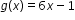can be expressed as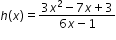, where the domain of h(x) is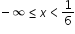and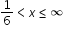.
MA.912.F.3.6: Determine whether an inverse function exists by analyzing tables, graphs and equations.
MA.912.FL.1.1: Extend previous knowledge of operations of fractions, percentages and decimals to solve real-world problems involving money and business.
 Clarifications:Clarification 1: Problems include discounts, markups, simple interest, tax, tips, fees, percent increase, percent decrease and percent error.
MA.912.FL.1.2: Extend previous knowledge of ratios and proportional relationships to solve real-world problems involving money and business.
 Examples:Example: A local grocery stores sells trail mix for \$1.75 per pound. If the grocery store spends \$0.82 on each pound of mix, how much will the store gain in gross profit if they sell 6.4 pounds in one day? Example: If Juan makes \$25.00 per hour and works 40 hours per week, what is his annual salary?
MA.912.FL.1.3: Solve real-world problems involving weighted averages using spreadsheets and other technology.
 Examples:Example: Kiko wants to buy a new refrigerator and decides on the following rating system: capacity 50%, water filter life 30% and capability with technology 20%. One refrigerator gets 8 (out of 10) for capacity, 6 for water filter life and 7 for capability with technology. Another refrigerator gets 9 for capacity, 4 for water filter life and 6 for capability with technology. Which refrigerator is best based on the rating system?
MA.912.FL.3.2: Solve real-world problems involving simple, compound and continuously compounded interest.
 Clarifications:Clarification 1: Within the Algebra 1 course, interest is limited to simple and compound.

 Examples:Example: Find the amount of money on deposit at the end of 5 years if you started with \$500 and it was compounded quarterly at 6% interest per year. Example: Joe won \$25,000 on a lottery scratch-off ticket. How many years will it take at 6% interest compounded yearly for his money to double?
MA.912.FL.3.4: Explain the relationship between simple interest and linear growth. Explain the relationship between compound interest and exponential growth and the relationship between continuously compounded interest and exponential growth.
 Clarifications:Clarification 1: Within the Algebra 1 course, exponential growth is limited to compound interest.
MA.912.GR.1.1: Prove relationships and theorems about lines and angles. Solve mathematical and real-world problems involving postulates, relationships and theorems of lines and angles.
 Clarifications:Clarification 1: Postulates, relationships and theorems include vertical angles are congruent; when a transversal crosses parallel lines, the consecutive angles are supplementary and alternate (interior and exterior) angles and corresponding angles are congruent; points on a perpendicular bisector of a line segment are exactly those equidistant from the segment’s endpoints.Clarification 2: Instruction includes constructing two-column proofs, pictorial proofs, paragraph and narrative proofs, flow chart proofs or informal proofs.Clarification 3: Instruction focuses on helping a student choose a method they can use reliably.
MA.912.GR.1.2: Prove triangle congruence or similarity using Side-Side-Side, Side-Angle-Side, Angle-Side-Angle, Angle-Angle-Side, Angle-Angle and Hypotenuse-Leg.
 Clarifications:Clarification 1: Instruction includes constructing two-column proofs, pictorial proofs, paragraph and narrative proofs, flow chart proofs or informal proofs.Clarification 2: Instruction focuses on helping a student choose a method they can use reliably.
MA.912.GR.1.3: Prove relationships and theorems about triangles. Solve mathematical and real-world problems involving postulates, relationships and theorems of triangles.
 Clarifications:Clarification 1: Postulates, relationships and theorems include measures of interior angles of a triangle sum to 180°; measures of a set of exterior angles of a triangle sum to 360°; triangle inequality theorem; base angles of isosceles triangles are congruent; the segment joining midpoints of two sides of a triangle is parallel to the third side and half the length; the medians of a triangle meet at a point.Clarification 2: Instruction includes constructing two-column proofs, pictorial proofs, paragraph and narrative proofs, flow chart proofs or informal proofs.Clarification 3: Instruction focuses on helping a student choose a method they can use reliably.
MA.912.GR.1.4: Prove relationships and theorems about parallelograms. Solve mathematical and real-world problems involving postulates, relationships and theorems of parallelograms.
 Clarifications:Clarification 1: Postulates, relationships and theorems include opposite sides are congruent, consecutive angles are supplementary, opposite angles are congruent, the diagonals of a parallelogram bisect each other, and rectangles are parallelograms with congruent diagonals.Clarification 2: Instruction includes constructing two-column proofs, pictorial proofs, paragraph and narrative proofs, flow chart proofs or informal proofs.Clarification 3: Instruction focuses on helping a student choose a method they can use reliably.
MA.912.GR.1.5: Prove relationships and theorems about trapezoids. Solve mathematical and real-world problems involving postulates, relationships and theorems of trapezoids.
 Clarifications:Clarification 1: Postulates, relationships and theorems include the Trapezoid Midsegment Theorem and for isosceles trapezoids: base angles are congruent, opposite angles are supplementary and diagonals are congruent.Clarification 2: Instruction includes constructing two-column proofs, pictorial proofs, paragraph and narrative proofs, flow chart proofs or informal proofs.Clarification 3: Instruction focuses on helping a student choose a method they can use reliably.
MA.912.GR.1.6: Solve mathematical and real-world problems involving congruence or similarity in two-dimensional figures.
 Clarifications:Clarification 1: Instruction includes demonstrating that two-dimensional figures are congruent or similar based on given information.
MA.912.GR.2.1: Given a preimage and image, describe the transformation and represent the transformation algebraically using coordinates.
 Clarifications:Clarification 1: Instruction includes the connection of transformations to functions that take points in the plane as inputs and give other points in the plane as outputs.Clarification 2: Transformations include translations, dilations, rotations and reflections described using words or using coordinates.Clarification 3: Within the Geometry course, rotations are limited to 90°, 180° and 270° counterclockwise or clockwise about the center of rotation, and the centers of rotations and dilations are limited to the origin or a point on the figure.

 Examples:Example: Given a triangle whose vertices have the coordinates (-3,4), (2,1.7) and (-0.4,-3). If this triangle is reflected across the y-axis the transformation can be described using coordinates as (x,y)→(-x,y) resulting in the image whose vertices have the coordinates (3,4), (-2,1.7) and (0.4,-3).
MA.912.GR.2.2: Identify transformations that do or do not preserve distance.
 Clarifications:Clarification 1: Transformations include translations, dilations, rotations and reflections described using words or using coordinates.Clarification 2: Instruction includes recognizing that these transformations preserve angle measure.
MA.912.GR.2.3: Identify a sequence of transformations that will map a given figure onto itself or onto another congruent or similar figure.
 Clarifications:Clarification 1: Transformations include translations, dilations, rotations and reflections described using words or using coordinates.Clarification 2: Within the Geometry course, figures are limited to triangles and quadrilaterals and rotations are limited to 90°, 180° and 270° counterclockwise or clockwise about the center of rotation.Clarification 3: Instruction includes the understanding that when a figure is mapped onto itself using a reflection, it occurs over a line of symmetry.
MA.912.GR.2.5: Given a geometric figure and a sequence of transformations, draw the transformed figure on a coordinate plane.
 Clarifications:Clarification 1: Transformations include translations, dilations, rotations and reflections described using words or using coordinates.Clarification 2: Instruction includes two or more transformations.
MA.912.GR.2.6: Apply rigid transformations to map one figure onto another to justify that the two figures are congruent.
 Clarifications:Clarification 1: Instruction includes showing that the corresponding sides and the corresponding angles are congruent.
MA.912.GR.2.8: Apply an appropriate transformation to map one figure onto another to justify that the two figures are similar.
 Clarifications:Clarification 1: Instruction includes showing that the corresponding sides are proportional, and the corresponding angles are congruent.
MA.912.GR.3.1: Determine the weighted average of two or more points on a line.
 Clarifications:Clarification 1: Instruction includes using a number line and determining how changing the weights moves the weighted average of points on the number line.
MA.912.GR.3.2: Given a mathematical context, use coordinate geometry to classify or justify definitions, properties and theorems involving circles, triangles or quadrilaterals.
 Clarifications:Clarification 1: Instruction includes using the distance or midpoint formulas and knowledge of slope to classify or justify definitions, properties and theorems.

 Examples:Example: Given Triangle ABC has vertices located at (-2,2), (3,3) and (1,-3), respectively, classify the type of triangle ABC is.Example: If a square has a diagonal with vertices (-1,1) and (-4,-3), find the coordinate values of the vertices of the other diagonal and show that the two diagonals are perpendicular.
MA.912.GR.3.3: Use coordinate geometry to solve mathematical and real-world geometric problems involving lines, circles, triangles and quadrilaterals.
 Clarifications:Clarification 1: Problems involving lines include the coordinates of a point on a line segment including the midpoint.Clarification 2: Problems involving circles include determining points on a given circle and finding tangent lines.Clarification 3: Problems involving triangles include median and centroid.Clarification 4: Problems involving quadrilaterals include using parallel and perpendicular slope criteria.

 Examples:Example: The line x+2y=10 is tangent to a circle whose center is located at (2,-1). Find the tangent point and a second tangent point of a line with the same slope as the given line.Example: Given M(-4,7) and N(12,-1),find the coordinates of point P on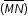so that P partitionsin the ratio 2:3.
MA.912.GR.3.4: Use coordinate geometry to solve mathematical and real-world problems on the coordinate plane involving perimeter or area of polygons.
 Examples:Example: A new community garden has four corners. Starting at the first corner and working counterclockwise, the second corner is 200 feet east, the third corner is 150 feet north of the second corner and the fourth corner is 100 feet west of the third corner. Represent the garden in the coordinate plane, and determine how much fence is needed for the perimeter of the garden and determine the total area of the garden.
MA.912.GR.4.1: Identify the shapes of two-dimensional cross-sections of three-dimensional figures.
 Clarifications:Clarification 1: Instruction includes the use of manipulatives and models to visualize cross-sections.Clarification 2: Instruction focuses on cross-sections of right cylinders, right prisms, right pyramids and right cones that are parallel or perpendicular to the base.
MA.912.GR.4.2: Identify three-dimensional objects generated by rotations of two-dimensional figures.
 Clarifications:Clarification 1: The axis of rotation must be within the same plane but outside of the given two-dimensional figure.
MA.912.GR.4.3: Extend previous understanding of scale drawings and scale factors to determine how dilations affect the area of two-dimensional figures and the surface area or volume of three-dimensional figures.
 Examples:Example: Mike is having a graduation party and wants to make sure he has enough pizza. Which option would provide more pizza for his guests: one 12-inch pizza or three 6-inch pizzas?
MA.912.GR.4.4: Solve mathematical and real-world problems involving the area of two-dimensional figures.
 Clarifications:Clarification 1: Instruction includes concepts of population density based on area.

 Examples:Example: A town has 23 city blocks, each of which has dimensions of 1 quarter mile by 1 quarter mile, and there are 4500 people in the town. What is the population density of the town?
MA.912.GR.4.5: Solve mathematical and real-world problems involving the volume of three-dimensional figures limited to cylinders, pyramids, prisms, cones and spheres.
 Clarifications:Clarification 1: Instruction includes concepts of density based on volume. Clarification 2: Instruction includes using Cavalieri’s Principle to give informal arguments about the formulas for the volumes of right and non-right cylinders, pyramids, prisms and cones.

 Examples:Example: A cylindrical swimming pool is filled with water and has a diameter of 10 feet and height of 4 feet. If water weighs 62.4 pounds per cubic foot, what is the total weight of the water in a full tank to the nearest pound?
MA.912.GR.4.6: Solve mathematical and real-world problems involving the surface area of three-dimensional figures limited to cylinders, pyramids, prisms, cones and spheres.
MA.912.GR.5.1: Construct a copy of a segment or an angle.
 Clarifications:Clarification 1: Instruction includes using compass and straightedge, string, reflective devices, paper folding or dynamic geometric software.
MA.912.GR.5.2: Construct the bisector of a segment or an angle, including the perpendicular bisector of a line segment.
 Clarifications:Clarification 1: Instruction includes using compass and straightedge, string, reflective devices, paper folding or dynamic geometric software.
MA.912.GR.5.3: Construct the inscribed and circumscribed circles of a triangle.
 Clarifications:Clarification 1: Instruction includes using compass and straightedge, string, reflective devices, paper folding or dynamic geometric software.
MA.912.GR.5.4: Construct a regular polygon inscribed in a circle. Regular polygons are limited to triangles, quadrilaterals and hexagons.
 Clarifications:Clarification 1: When given a circle, the center must be provided.Clarification 2: Instruction includes using compass and straightedge, string, reflective devices, paper folding or dynamic geometric software.
MA.912.GR.5.5: Given a point outside a circle, construct a line tangent to the circle that passes through the given point.
 Clarifications:Clarification 1: When given a circle, the center must be provided.Clarification 2: Instruction includes using compass and straightedge, string, reflective devices, paper folding or dynamic geometric software.
MA.912.GR.6.1: Solve mathematical and real-world problems involving the length of a secant, tangent, segment or chord in a given circle.
 Clarifications:Clarification 1: Problems include relationships between two chords; two secants; a secant and a tangent; and the length of the tangent from a point to a circle.
MA.912.GR.6.2: Solve mathematical and real-world problems involving the measures of arcs and related angles.
 Clarifications:Clarification 1: Within the Geometry course, problems are limited to relationships between inscribed angles; central angles; and angles formed by the following intersections: a tangent and a secant through the center, two tangents, and a chord and its perpendicular bisector.
MA.912.GR.6.3: Solve mathematical problems involving triangles and quadrilaterals inscribed in a circle.
 Clarifications:Clarification 1: Instruction includes cases in which a triangle inscribed in a circle has a side that is the diameter.
MA.912.GR.6.4: Solve mathematical and real-world problems involving the arc length and area of a sector in a given circle.
 Clarifications:Clarification 1: Instruction focuses on the conceptual understanding that for a given angle measure the length of the intercepted arc is proportional to the radius, and for a given radius the length of the intercepted arc is proportional is the angle measure.
MA.912.GR.7.2: Given a mathematical or real-world context, derive and create the equation of a circle using key features.
 Clarifications:Clarification 1: Instruction includes using the Pythagorean Theorem and completing the square. Clarification 2: Within the Geometry course, key features are limited to the radius, diameter and the center.
MA.912.GR.7.3: Graph and solve mathematical and real-world problems that are modeled with an equation of a circle. Determine and interpret key features in terms of the context.
 Clarifications:Clarification 1: Key features are limited to domain, range, eccentricity, center and radius.Clarification 2: Instruction includes representing the domain and range with inequality notation, interval notation or set-builder notation.Clarification 3: Within the Geometry course, notations for domain and range are limited to inequality and set-builder.
MA.912.NSO.1.1: Extend previous understanding of the Laws of Exponents to include rational exponents. Apply the Laws of Exponents to evaluate numerical expressions and generate equivalent numerical expressions involving rational exponents.
 Clarifications:Clarification 1: Instruction includes the use of technology when appropriate. Clarification 2: Refer to the K-12 Formulas (Appendix E) for the Laws of Exponents. Clarification 3: Instruction includes converting between expressions involving rational exponents and expressions involving radicals.Clarification 4:Within the Mathematics for Data and Financial Literacy course, it is not the expectation to generate equivalent numerical expressions.
MA.912.NSO.1.2: Generate equivalent algebraic expressions using the properties of exponents.
 Examples:The expression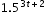is equivalent to the expression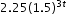which is equivalent to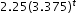.
MA.912.NSO.1.4: Apply previous understanding of operations with rational numbers to add, subtract, multiply and divide numerical radicals.
 Clarifications:Clarification 1: Within the Algebra 1 course, expressions are limited to a single arithmetic operation involving two square roots or two cube roots.

 Examples:Algebra 1 Example: The expression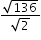is equivalent to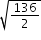which is equivalent to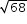which is equivalent to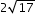.
MA.912.NSO.1.6: Given a numerical logarithmic expression, evaluate and generate equivalent numerical expressions using the properties of logarithms or exponents.
 Clarifications:Clarification 1: Within the Mathematics for Data and Financial Literacy Honors course, problem types focus on money and business.
MA.912.NSO.2.2: Represent addition, subtraction, multiplication and conjugation of complex numbers geometrically on the complex plane.
MA.912.T.1.1: Define trigonometric ratios for acute angles in right triangles.
 Clarifications: Clarification 1: Instruction includes using the Pythagorean Theorem and using similar triangles to demonstrate that trigonometric ratios stay the same for similar right triangles.Clarification 2: Within the Geometry course, instruction includes using the coordinate plane to make connections to the unit circle.Clarification 3: Within the Geometry course, trigonometric ratios are limited to sine, cosine and tangent.
MA.912.T.1.2: Solve mathematical and real-world problems involving right triangles using trigonometric ratios and the Pythagorean Theorem.
 Clarifications:Clarification 1: Instruction includes procedural fluency with the relationships of side lengths in special right triangles having angle measures of 30°-60°-90° and 45°-45°-90°.
MA.K12.MTR.1.1: Actively participate in effortful learning both individually and collectively.

Mathematicians who participate in effortful learning both individually and with others:

• Analyze the problem in a way that makes sense given the task.
• Build perseverance by modifying methods as needed while solving a challenging task.
• Stay engaged and maintain a positive mindset when working to solve tasks.
• Help and support each other when attempting a new method or approach.

 Clarifications:Teachers who encourage students to participate actively in effortful learning both individually and with others: Cultivate a community of growth mindset learners.  Foster perseverance in students by choosing tasks that are challenging. Develop students’ ability to analyze and problem solve. Recognize students’ effort when solving challenging problems.
MA.K12.MTR.2.1: Demonstrate understanding by representing problems in multiple ways.

Mathematicians who demonstrate understanding by representing problems in multiple ways:

• Build understanding through modeling and using manipulatives.
• Represent solutions to problems in multiple ways using objects, drawings, tables, graphs and equations.
• Progress from modeling problems with objects and drawings to using algorithms and equations.
• Express connections between concepts and representations.
• Choose a representation based on the given context or purpose.
 Clarifications:Teachers who encourage students to demonstrate understanding by representing problems in multiple ways: Help students make connections between concepts and representations.Provide opportunities for students to use manipulatives when investigating concepts.Guide students from concrete to pictorial to abstract representations as understanding progresses.Show students that various representations can have different purposes and can be useful in different situations.
MA.K12.MTR.3.1: Complete tasks with mathematical fluency.

Mathematicians who complete tasks with mathematical fluency:

• Select efficient and appropriate methods for solving problems within the given context.
• Maintain flexibility and accuracy while performing procedures and mental calculations.
• Complete tasks accurately and with confidence.
• Adapt procedures to apply them to a new context.
• Use feedback to improve efficiency when performing calculations.
 Clarifications:Teachers who encourage students to complete tasks with mathematical fluency:Provide students with the flexibility to solve problems by selecting a procedure that allows them to solve efficiently and accurately.Offer multiple opportunities for students to practice efficient and generalizable methods.Provide opportunities for students to reflect on the method they used and determine if a more efficient method could have been used.
MA.K12.MTR.4.1: Engage in discussions that reflect on the mathematical thinking of self and others.

Mathematicians who engage in discussions that reflect on the mathematical thinking of self and others:

• Communicate mathematical ideas, vocabulary and methods effectively.
• Analyze the mathematical thinking of others.
• Compare the efficiency of a method to those expressed by others.
• Recognize errors and suggest how to correctly solve the task.
• Justify results by explaining methods and processes.
• Construct possible arguments based on evidence.
 Clarifications:Teachers who encourage students to engage in discussions that reflect on the mathematical thinking of self and others:Establish a culture in which students ask questions of the teacher and their peers, and error is an opportunity for learning.Create opportunities for students to discuss their thinking with peers.Select, sequence and present student work to advance and deepen understanding of correct and increasingly efficient methods.Develop students’ ability to justify methods and compare their responses to the responses of their peers.
MA.K12.MTR.5.1: Use patterns and structure to help understand and connect mathematical concepts.

Mathematicians who use patterns and structure to help understand and connect mathematical concepts:

• Focus on relevant details within a problem.
• Create plans and procedures to logically order events, steps or ideas to solve problems.
• Decompose a complex problem into manageable parts.
• Relate previously learned concepts to new concepts.
• Look for similarities among problems.
• Connect solutions of problems to more complicated large-scale situations.
 Clarifications:Teachers who encourage students to use patterns and structure to help understand and connect mathematical concepts:Help students recognize the patterns in the world around them and connect these patterns to mathematical concepts.Support students to develop generalizations based on the similarities found among problems.Provide opportunities for students to create plans and procedures to solve problems.Develop students’ ability to construct relationships between their current understanding and more sophisticated ways of thinking.
MA.K12.MTR.6.1: Assess the reasonableness of solutions.

Mathematicians who assess the reasonableness of solutions:

• Estimate to discover possible solutions.
• Use benchmark quantities to determine if a solution makes sense.
• Check calculations when solving problems.
• Verify possible solutions by explaining the methods used.
• Evaluate results based on the given context.
 Clarifications:Teachers who encourage students to assess the reasonableness of solutions:Have students estimate or predict solutions prior to solving.Prompt students to continually ask, “Does this solution make sense? How do you know?”Reinforce that students check their work as they progress within and after a task.Strengthen students’ ability to verify solutions through justifications.
MA.K12.MTR.7.1: Apply mathematics to real-world contexts.

Mathematicians who apply mathematics to real-world contexts:

• Connect mathematical concepts to everyday experiences.
• Use models and methods to understand, represent and solve problems.
• Perform investigations to gather data or determine if a method is appropriate. • Redesign models and methods to improve accuracy or efficiency.
 Clarifications:Teachers who encourage students to apply mathematics to real-world contexts:Provide opportunities for students to create models, both concrete and abstract, and perform investigations.Challenge students to question the accuracy of their models and methods.Support students as they validate conclusions by comparing them to the given situation.Indicate how various concepts can be applied to other disciplines.
ELA.K12.EE.1.1: Cite evidence to explain and justify reasoning.
 Clarifications:K-1 Students include textual evidence in their oral communication with guidance and support from adults. The evidence can consist of details from the text without naming the text. During 1st grade, students learn how to incorporate the evidence in their writing.2-3 Students include relevant textual evidence in their written and oral communication. Students should name the text when they refer to it. In 3rd grade, students should use a combination of direct and indirect citations.4-5 Students continue with previous skills and reference comments made by speakers and peers. Students cite texts that they’ve directly quoted, paraphrased, or used for information. When writing, students will use the form of citation dictated by the instructor or the style guide referenced by the instructor. 6-8 Students continue with previous skills and use a style guide to create a proper citation.9-12 Students continue with previous skills and should be aware of existing style guides and the ways in which they differ.
 Clarifications:See Text Complexity for grade-level complexity bands and a text complexity rubric.
ELA.K12.EE.3.1: Make inferences to support comprehension.
 Clarifications:Students will make inferences before the words infer or inference are introduced. Kindergarten students will answer questions like “Why is the girl smiling?” or make predictions about what will happen based on the title page. Students will use the terms and apply them in 2nd grade and beyond.
ELA.K12.EE.4.1: Use appropriate collaborative techniques and active listening skills when engaging in discussions in a variety of situations.
 Clarifications:In kindergarten, students learn to listen to one another respectfully.In grades 1-2, students build upon these skills by justifying what they are thinking. For example: “I think ________ because _______.” The collaborative conversations are becoming academic conversations.In grades 3-12, students engage in academic conversations discussing claims and justifying their reasoning, refining and applying skills. Students build on ideas, propel the conversation, and support claims and counterclaims with evidence.
ELA.K12.EE.5.1: Use the accepted rules governing a specific format to create quality work.
 Clarifications:Students will incorporate skills learned into work products to produce quality work. For students to incorporate these skills appropriately, they must receive instruction. A 3rd grade student creating a poster board display must have instruction in how to effectively present information to do quality work.
ELA.K12.EE.6.1: Use appropriate voice and tone when speaking or writing.
 Clarifications:In kindergarten and 1st grade, students learn the difference between formal and informal language. For example, the way we talk to our friends differs from the way we speak to adults. In 2nd grade and beyond, students practice appropriate social and academic language to discuss texts.
ELD.K12.ELL.MA.1: English language learners communicate information, ideas and concepts necessary for academic success in the content area of Mathematics.

## General Course Information and Notes

### VERSION DESCRIPTION

This course supports students who need additional instruction in foundational mathematics skills as it relates to core instruction. Instruction will use explicit, systematic, and sequential approaches to mathematics instruction addressing all strands including number sense & operations, algebraic reasoning, functions, geometric reasoning and data analysis & probability. Teachers will use the listed benchmarks that correspond to each students’ needs.

Effective instruction matches instruction to the need of the students in the group and provides multiple opportunities to practice the skill and receive feedback. The additional time allotted for this course is in addition to core instruction. The intervention includes materials and strategies designed to supplement core instruction.

### GENERAL NOTES

Florida’s Benchmarks for Excellent Student Thinking (B.E.S.T.) Standards
This course includes Florida’s B.E.S.T. ELA Expectations (EE) and Mathematical Thinking and Reasoning Standards (MTRs) for students. Florida educators should intentionally embed these standards within the content and their instruction as applicable. For guidance on the implementation of the EEs and MTRs, please visit https://www.cpalms.org/Standards/BEST_Standards.aspx and select the appropriate B.E.S.T. Standards package.

English Language Development ELD Standards Special Notes Section:

Teachers are required to provide listening, speaking, reading and writing instruction that allows English language learners (ELL) to communicate information, ideas and concepts for academic success in the content area of Mathematics. For the given level of English language proficiency and with visual, graphic, or interactive support, students will interact with grade level words, expressions, sentences and discourse to process or produce language necessary for academic success. The ELD standard should specify a relevant content area concept or topic of study chosen by curriculum developers and teachers which maximizes an ELL’s need for communication and social skills. To access an ELL supporting document which delineates performance definitions and descriptors, please click on the following link: https://cpalmsmediaprod.blob.core.windows.net/uploads/docs/standards/eld/ma.pdf

### General Information

 Course Number: 1200400 Course Path: Section: Grades PreK to 12 Education Courses > Grade Group: Grades 9 to 12 and Adult Education Courses > Subject: Mathematics > SubSubject: Remedial > Abbreviated Title: FDN SKILLS MATH 9-12 Number of Credits: Multiple Credit (more than 1 credit) Course Attributes: Florida Standards Course Course Type: Elective Course Course Level: 2 Course Status: State Board Approved Grade Level(s): 9,10,11,12

#### Educator Certifications

 MathXL for SchoolPearson - Savvas Learning Company LLC, formerly known as Pearson K12 Learning LLC. - 1 - 2009